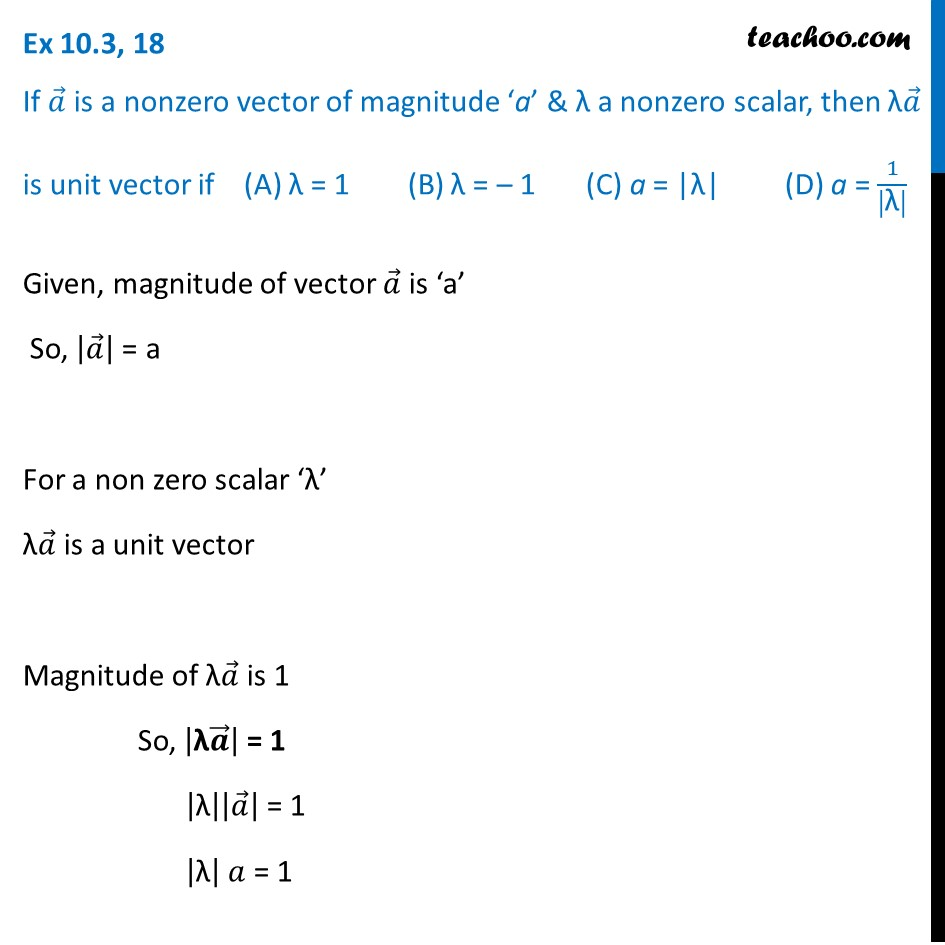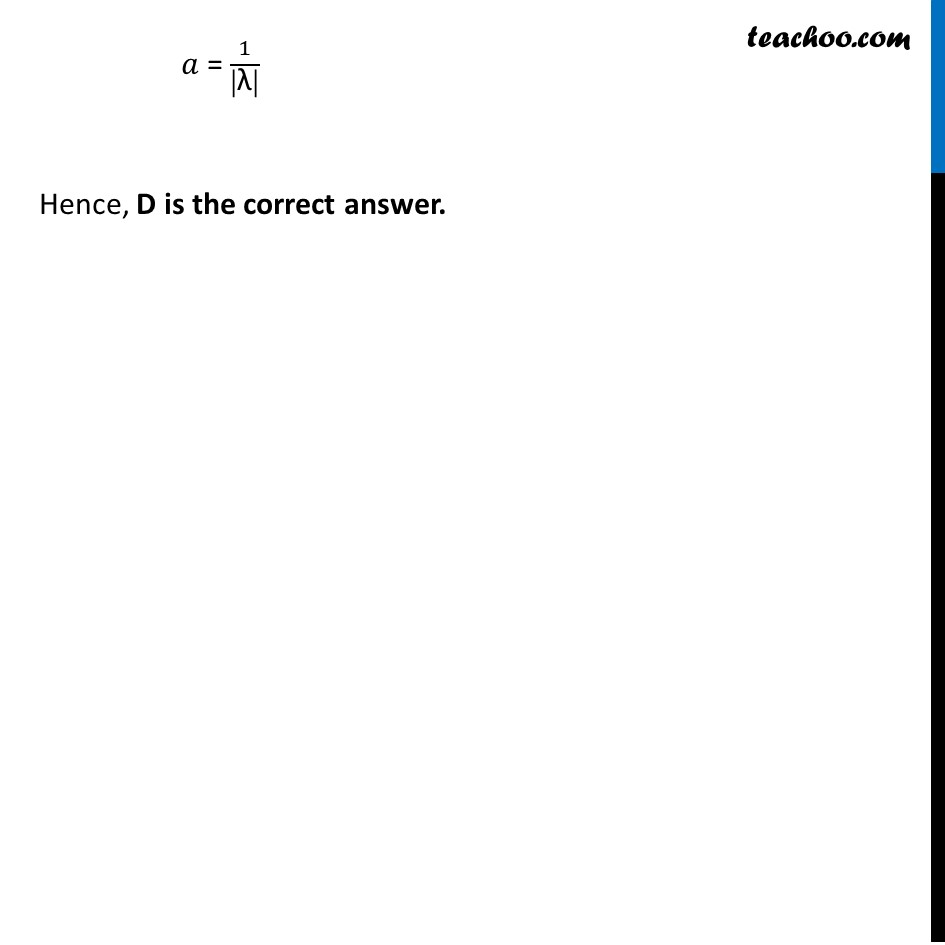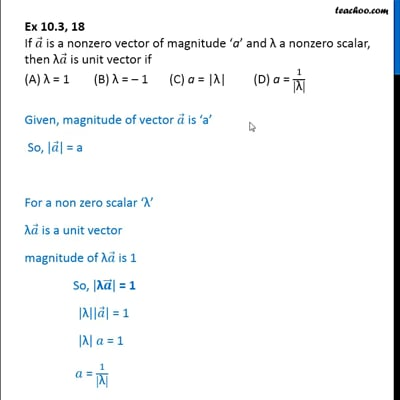Unit vector

Chapter 10 Class 12 Vector Algebra
Concept wiseThis video is only available for Teachoo black users

Introducing your new favourite teacher - Teachoo Black, at only ₹83 per month

### Transcript

Ex 10.3, 18 If 𝑎 ⃗ is a nonzero vector of magnitude ‘a’ & λ a nonzero scalar, then λ𝑎 ⃗ is unit vector if (A) λ = 1 (B) λ = – 1 (C) a = |λ| (D) a = 1/|"λ" | Given, magnitude of vector 𝑎 ⃗ is ‘a’ So, |𝑎 ⃗ | = a For a non zero scalar ‘λ’ λ𝑎 ⃗ is a unit vector Magnitude of λ𝑎 ⃗ is 1 So, |"λ" 𝒂 ⃗ | = 1 |"λ" ||𝑎 ⃗ | = 1 |"λ" | 𝑎 = 1 𝑎 = 1/|"λ" | Hence, D is the correct answer.